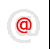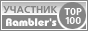Apache-Talk @lexa.ruInet-Admins @info.east.ruSecurity-alerts @yandex-team.runginx-ru @sysoev.ruđéűéôĺđéóříááňčé÷ :: Filmscanners
Filmscanners mailing list archive (filmscanners@halftone.co.uk)

[filmscanners] Re: Density vs Dynamic range

Hi Austin,

This HAS to be a problem of semantics!   You are waging a war on so many

You say:
"Simply put, dynamic range is the (max value - min value) divided by noise."

But NO - dynamic range is max value - min value (when expressed as a
difference) ***OR*** max value divided by min value (as a ratio).

Since we all agree that min value = noise level, then you are including
noise level twice in the equation/definition and thus confusing things
immeasurably.  You say that

DR = (max - min) / noise
= (max - noise) / noise

But this is just not true. And I know that you know it is not true because
you say so at other times, and your references say so, which is why I think
we have a semantic problem here.

You say:
>the Dynamic Range equation out of "Digital Signal Processing in VLSI":
>DR (dB) = 10log10(largest signal/smallest discernable signal) or:
>3.6 is equal to 10**3.6 or 3981, and .2 is 10**.2 or 1.5849...
>SO...
>DR (in Bells, not DECI-Bells) = log10(3981/1.5849)
>Which, is 3.4 ;-)

This is correct.  There it is - you say DR = max/min which is the ratio
expression of dynamic range.  NOT (max-min)/min.  Just max/min.  That is
the ratio version of the definition of DR.  Dynamic range is a range, not a
number of steps, by definition.  It happens that the OPTIMUM number of
steps is equal to the number that is the DR expressed as a ratio to
one.  But this is not the same as saying that the DR is defined as the
number of steps.  It is not, it is simply a RANGE, usually expressed as a
ratio.

Simple example.  Signal with 3mW of noise and 30mW max.  The dynamic range
from first principles is simply "3 to 30mW".  There it is -  as a real
basic range.  "3 to 30mW".

This can be expressed as a single figure in two ways - as a
***difference*** or as a ***ratio***.  As a difference, the DR is 27mW, but
this is difficult to use because you have to know the upper or lower limit,
so it is really "27mW with a top of 30mW" or "27 mW with a bottom of 3mW".

For this reason and because the range is so great normally, obviously we
usually express it as a ratio.  In this case the DR as a ratio is 30/3 or
10.  The Dynamic range is 10, this number expresses a RANGE as a ratio
between largest and smallest. You can dB or B it if you like.

So, again, the dynamic range is ***either***    MAX -
MIN  ***OR  ***  MAX/MIN  depending on which is most useful to you.  The
standard in signal theory as you know is as you quoted it above - MAX/MIN,
or at least the log form of that.

But it is NOT  ...      (MAX - MIN) / MIN which is what you keep saying and
is at the core of the problem here.

The difference between the two points of view is twofold, one is this
"equation difference" and the other is the definitional difference where
you keep equivalencing "number of steps" with "dynamic range" just because
the OPTIMUM number of steps happens to have the same number as the dynamic
range when expressed as a ratio to one.

Dynamic range is dB (usually), while number of steps is n, a number, not a
ratio.  You get the OPTIMUM number of steps n from the dB ratio, but they
are NOT the same thing, despite being the same actual number when expressed
in one particular way.

As for definition of dynamic, here is where I really do not agree with
you.  Maybe radar and hi-fi and other areas are different from scanners,
but I don't think so.

"Dynamic" refers to what can be handled by the electronic system at one
particular time, as opposed to what can be handled under different (gain or
ALC (auto level control)) conditions.

In my radar example, the dynamic range might be 50dB.  But the total input
range that can be handled might be 90dB because you can choose to put more
attenuation before the dynamic range limiting part of the circuit to reduce
large signals to what can be handled within that 50 dB dynamic range.  In a
radar this is done at the beginning of each range sweep so that you can see
what is close as well as what is far on the same viewing system which can
NEVER have a dynamic range of more than around 20dB just because that is
what the eye can see.  (we are neglecting digital processing, but the
principle is the same).

Lastly,

Julian R. said:
> > So the optimum number of bits is decided by:
> >
> > num coded levels =  max level divided by optimum digital step size
> >                  = max level divided by noise level.
Austin replied:
>Er, that is EXACTLY THE SAME FRIGGING EQUATION I USED.  GRRRR!!!!!
>...But yet here, you are DIVIDING IT BY NOISE.  What am I missing here?

What you are missing in terms of what I am trying to say is <<< what is on
the left of the equals sign>>> (POINT 1).  There is also one term different
in the equations we use (POINT 2).

POINT 1:  You would put dynamic range on the left of the equals sign and I
would not.  I put "optimum number of levels".  As we keep realising, the
optimum number of levels EQUALS the dynamic range expressed as a ratio to
one, but that is not saying they are the same thing.

POINT 2: You in fact do say this at times (I.e. max level divided by noise
level), but at other times you say  (max level - min level) divided by
noise level.  These are different, not so much in the number that results
because the variation is usually small, but in terms of the MEANING and
DEFINITION that it implies.

Are we converging?

Julian R

----------------------------------------------------------------------------------------
Unsubscribe by mail to listserver@halftone.co.uk, with 'unsubscribe
filmscanners'
or 'unsubscribe filmscanners_digest' (as appropriate) in the message title or
bodyCopyright © Lexa Software, 1996-2009.